# 后缀数组详解

## 前置知识

### 后缀## 倍增法

$sa[i]$：排名为$i$的后缀的位置

$rak[i]$：从第$i$个位置开始的后缀的排名，下文为了叙述方便，把从第$i$个位置开始的后缀简称为后缀$i$

$tp[i]$：基数排序的第二关键字，意义与$sa$一样，即第二关键字排名为$i$的后缀的位置

$tax[i]$：$i$号元素出现了多少次。辅助基数排序

$s$：字符串，$s[i]$表示字符串中第$i$个字符串

$rak[sa[i]]=i$

$sa[rak[i]]=i$$tp[i]$表示的是第二关键字中排名为$i$的后缀的位置，$rak$表示的是上一轮中第$i$个后缀的排名。

## 过程详解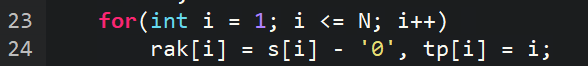$M$：字符集的大小，基数排序时会用到。不理解也没关系

$p$：排名的多少(有几个不同的后缀)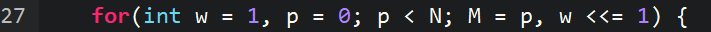$w$表示倍增的长度，当各个排名都不相同时，我们便可以退出循环。

$M=p$是对基数排序的优化，因为字符集大小就是排名的个数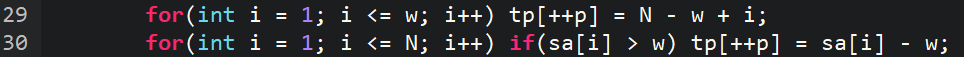（注意此图的边界与代码中有区别，原因是代码中的$w$表示我们已经得到了长度为$w$的结果，现在正要去更新长度为$2w$的结果）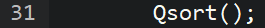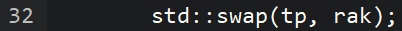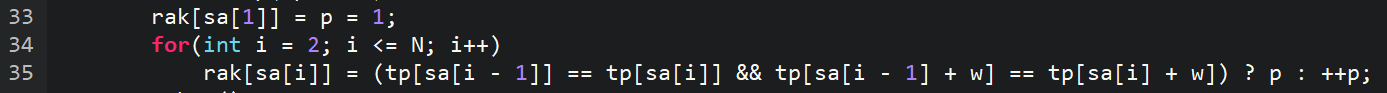然后愉快的输出就可以啦！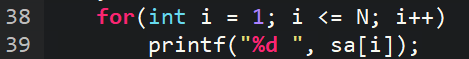#include<cstdio>
#include<cstring>
#include<algorithm>
const int MAXN = 1e6 + 10;
using namespace std;
char s[MAXN];
int N, M, rak[MAXN], sa[MAXN], tax[MAXN], tp[MAXN];
void Debug() {
printf("*****************\n");
printf("下标"); for (int i = 1; i <= N; i++) printf("%d ", i);     printf("\n");
printf("sa  "); for (int i = 1; i <= N; i++) printf("%d ", sa[i]); printf("\n");
printf("rak "); for (int i = 1; i <= N; i++) printf("%d ", rak[i]); printf("\n");
printf("tp  "); for (int i = 1; i <= N; i++) printf("%d ", tp[i]); printf("\n");
}
void Qsort() {
for (int i = 0; i <= M; i++) tax[i] = 0;
for (int i = 1; i <= N; i++) tax[rak[i]]++;
for (int i = 1; i <= M; i++) tax[i] += tax[i - 1];
for (int i = N; i >= 1; i--) sa[ tax[rak[tp[i]]]-- ] = tp[i];
//这部分我的文章的末尾详细的说明了
}
void SuffixSort() {
M = 75;
for (int i = 1; i <= N; i++) rak[i] = s[i] - '0' + 1, tp[i] = i;
Qsort();
Debug();
for (int w = 1, p = 0; p < N; M = p, w <<= 1) {
//w:当前倍增的长度，w = x表示已经求出了长度为x的后缀的排名，现在要更新长度为2x的后缀的排名
//p表示不同的后缀的个数，很显然原字符串的后缀都是不同的，因此p = N时可以退出循环
p = 0;//这里的p仅仅是一个计数器000
for (int i = 1; i <= w; i++) tp[++p] = N - w + i;
for (int i = 1; i <= N; i++) if (sa[i] > w) tp[++p] = sa[i] - w; //这两句是后缀数组的核心部分，我已经画图说明
Qsort();//此时我们已经更新出了第二关键字，利用上一轮的rak更新本轮的sa
std::swap(tp, rak);//这里原本tp已经没有用了
rak[sa] = p = 1;
for (int i = 2; i <= N; i++)
rak[sa[i]] = (tp[sa[i - 1]] == tp[sa[i]] && tp[sa[i - 1] + w] == tp[sa[i] + w]) ? p : ++p;
//这里当两个后缀上一轮排名相同时本轮也相同，至于为什么大家可以思考一下
Debug();
}
for (int i = 1; i <= N; i++)
printf("%d ", sa[i]);
}
int main() {
scanf("%s", s + 1);
N = strlen(s + 1);
SuffixSort();
return 0;
}## 基数排序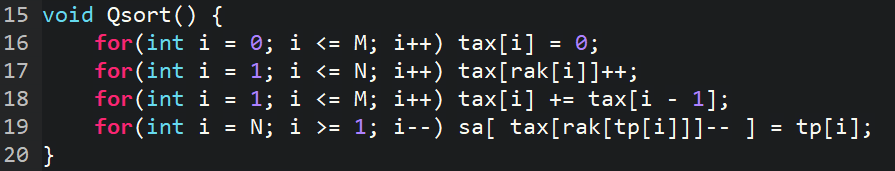$M$：字符集的大小，一共需要多少个桶

$tax$：元素出现的次数，在这里就是名次出现的次数

$sa[i]$：长度为$w$的后缀中，排名为$i$的后缀的位置

$rak[i]$：长度为$w$的后缀中，从第$i$个位置开始的后缀的排名

$tp[i]$：长度为$2w$的后缀中，第二关键字排名为$i$的后缀的位置

## height数组

$i$号后缀：从$i$开始的后缀

$lcp(x,y)$：字符串$x$与字符串$y$的最长公共前缀，在这里指$x$号后缀与与$y$号后缀的最长公共前缀

$height[i]$：$lcp(sa[i], sa[i - 1])$，即排名为$i$的后缀与排名为$i - 1$的后缀的最长公共前缀

$H[i]$：$height[rak[i]]$，即$i$号后缀与它前一名的后缀的最长公共前缀

update in 2019.3.28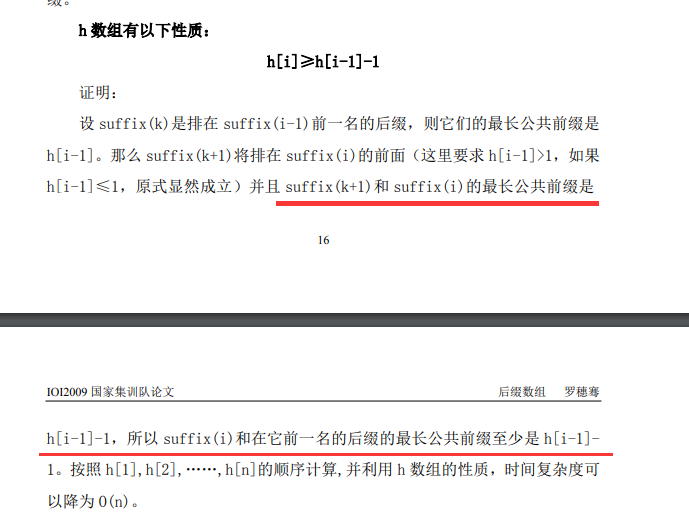void GetHeight() {
int j, k = 0;
for(int i = 1; i <= N; i++) {
if(k) k--;
int j = sa[rak[i] - 1];
while(s[i + k] == s[j + k]) k++;
Height[rak[i]] = k;
printf("%d\n", k);
}
}

## 经典应用

### 两个后缀的最大公共前缀

$lcp(x, y) = min(heigh[x-y])$， 用rmq维护，O(1)查询

Height数组里的最大值

## 后记

posted @ 2018-02-06 11:36  自为风月马前卒  阅读(10483)  评论(29编辑  收藏

……